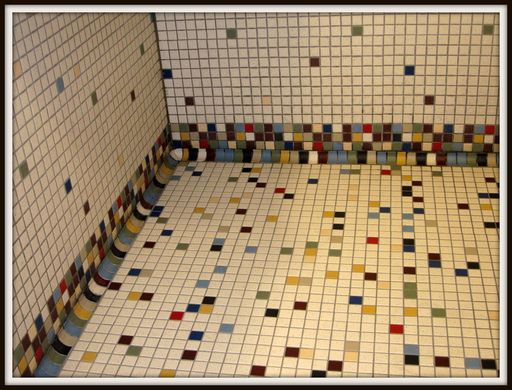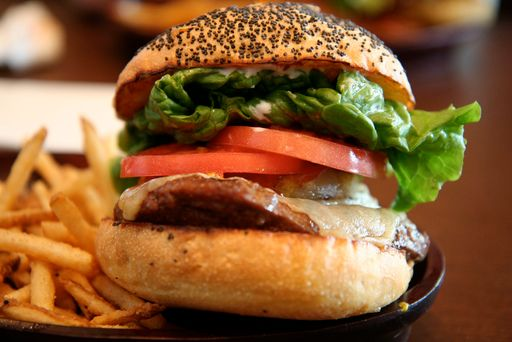Home Practice
For learners and parents For teachers and schools
Textbooks
Full catalogue
Pricing SupportLog in

We think you are located in United States. Is this correct?

# 1.5 Rounding

## 1.5 Rounding (EMGR)

### Rounding off according to the context (EMGS)

When we round off numbers, we need to be aware of the context of the problem. This will determine whether we round off, up or down.

When we round off to the nearest $$\text{10}$$, we follow the simple rule that numbers with units digits from $$\text{1}$$ to $$\text{4}$$ are rounded down to the lower ten, while numbers with units digits from $$\text{5}$$ to $$\text{9}$$ are rounded up to the higher ten.However, when we are working in some practical, real-life situations, we must think carefully about what the results of rounding off will be. In other words, the answer must be reasonable so that it is not only correct, but also makes sense in the situation.

For example, South Africa no longer has 1c and 2c coins, so shops need to round off the totals to a 5c value if customers are paying cash. Shops round down, rather than rounding off. So if your total is $$\text{R}\,\text{13,69}$$, you would pay $$\text{R}\,\text{13,65}$$ in cash. If you pay by credit or debit card however, the totals are not rounded off.

## Worked example 12: Rounding up and down

Answer the following questions and in each case explain why you would round up or down to get a reasonable answer.

1. Jacolene is catering for a group of $$\text{54}$$ people. The muffins are sold in packs of $$\text{8}$$. How many packs of muffins must she buy?2. A group of learners is going to the Maropeng Centre at the Cradle of Humankind. There are $$\text{232}$$ learners and teachers going on the outing. The school needs to hire buses and each bus can carry $$\text{50}$$ passengers.

1. How many buses should they hire?
2. How many empty seats will there be?
3. Ludwe is buying blinds for a large window in his home. Each blind is $$\text{100}$$ $$\text{cm}$$ wide. The window is $$\text{260}$$ $$\text{cm}$$ wide. How many blinds does he need?1. Jacolene has to decide whether to round up and buy $$\text{7} \times \text{8} = \text{56}$$ muffins, so that there will definitely be enough for each person to have one, or whether it is unlikely that everyone will want a muffin, in which case she can round down to $$\text{6} \times \text{8} =\text{48}$$ muffins.
1. To make sure that no learners are left behind, we have to round the number of seats up to the next $$\text{50}$$, so $$\text{232}$$ is rounded up to $$\text{250}$$, and there must be $$\text{5}$$ buses.
2. There will be $$\text{250}$$ - $$\text{232}$$ = $$\text{18}$$ empty seats.
2. In this situation, Ludwe will probably round up again, as it would be a problem to have a part of the window exposed, and more acceptable to have the blinds wider than the window width. So he would round up the total width of blinds to $$\text{300}$$ $$\text{cm}$$, so that there would be $$\text{3}$$ blinds in total.

## Rounding off in real-life situations

Exercise 1.7

Michael needs $$\text{1 245}$$ tiles to tile a bathroom. He can only buy tiles in packs of $$\text{75}$$.

Should he round the number of tiles up or down to see how many he should buy? Explain.

He should round up. If he rounds down he won't have enough tiles to cover the floor!

How many packs should he buy?$$\text{1 245} \div \text{75} = \text{16,6}$$. So he should buy $$\text{17}$$ packs.

A classroom wall is $$\text{750}$$ $$\text{cm}$$ long.

How many tables, each $$\text{120}$$ $$\text{cm}$$ long, will fit along the wall?

$$\text{750}\text{ cm} \div \text{120}\text{ cm} = \text{6,25}$$. So $$\text{6}$$ tables will fit along the wall.

How much space will be left over?

$$\text{6} \times \text{120}\text{ cm} = \text{720}\text{ cm}$$, so there will be $$\text{750}\text{ cm} - \text{720}\text{ cm}= \text{30}\text{ cm}$$ left over.

There are $$\text{231}$$ learners in a Grade 10 group. They each need an exercise book, which are sold in packs of $$\text{25}$$.

How many packs of books should be ordered?

$$\text{231} \div \text{25} = \text{9,24}$$. $$\text{10}$$ packs should be ordered.

How many spare exercise books will there be?

$$\text{10} \times \text{25} = \text{250}$$. $$\text{250}$$ - $$\text{231}$$ = $$\text{19}$$ spare books.

Julia needs to make $$\text{500}$$ hamburgers for a school function. Hamburger patties are sold in packets of $$\text{12}$$.

How many packets of patties should she buy?

$$\text{500} \div \text{12} = \text{41,67}$$. She should buy $$\text{42}$$ packs.

How many will be left over?$$\text{42} \times \text{12} = \text{504}$$. $$\text{504}$$ - $$\text{500}$$ = $$\text{4}$$ spare patties.

Car parking spaces should be $$\text{2,5}$$ $$\text{m}$$ wide. How many parking spaces should be painted in a car park which is $$\text{72}$$ $$\text{m}$$ wide?

$$\text{70} \div \text{2,5} = \text{28,8}$$. So $$\text{28}$$ parking spaces can be painted in the car park.